# Resistivity

Electrical resistivity (also known as specific electrical resistance) is a measure of how strongly a material opposes the flow of electric current. A low value of resistivity indicates a material that readily allows the movement of electrical charge. The SI unit of electrical resistivity is the ohm meter (Ω-m). Knowledge of the resistivity of various materials is useful for the choice of materials needed for manufacturing electrical and electronic components.

## Definitions

The electrical resistivity ρ (rho) of a material is given by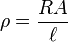$\rho=\frac{RA}{\ell}$

where

ρ is the static resistivity (measured in ohm meters, Ω-m);
R is the electrical resistance of a uniform specimen of the material (measured in ohms, Ω);$\ell$ is the length of the piece of material (measured in meters, m);
A is the cross-sectional area of the specimen (measured in square meters, m²).

Electrical resistivity can also be defined as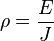$\rho={E \over J}$

where

E is the magnitude of the electric field (measured in volts per meter, V/m);
J is the magnitude of the current density (measured in amperes per square meter, A/m²).

Finally, electrical resistivity is also defined as the inverse of the conductivity σ (sigma), of the material, or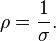$\rho = {1\over\sigma}.$

## Table of resistivities

This table shows the resistivity and temperature coefficient of various materials at 20 °C (68 °F)

Material Resistivity (Ω-m) at 20 °C Coefficient* Reference
Silver 1.59×10−8 .0038 
Copper 1.72×10−8 .0039 
Gold 2.44×10−8 .0034 
Aluminium 2.82×10−8 .0039 
Calcium 3.3x10-8
Tungsten 5.60×10−8 .0045 
Nickel 6.99×10−8 ?
Iron 1.0×10−7 .005 
Tin 1.09×10−7 .0045
Platinum 1.1×10−7 .00392 
Manganin 4.82×10−7 .000002 
Constantan 4.9×10−7 0.00001 
Mercury 9.8×10−7 .0009 
Nichrome 1.10×10−6 .0004 
Carbon 3.5×10−5 -.0005 
Germanium 4.6×10−1 -.048 
Silicon 6.40×102 -.075 
Glass 1010 to 1014 ? 
Hard rubber approx. 1013 ? 
Sulfur 1015 ? 
Paraffin 1017 ?
Quartz (fused) 7.5×1017 ? 
PET 1020 ?
Teflon 1022 to 1024 ?

*The numbers in this column increase or decrease the significand portion of the resistivity. For example, at 30°C (303.15 K), the resistivity of silver is 1.65×10−8. This is calculated as Δρ = α ΔT ρo where ρo is the resistivity at 20°C and α is the temperature coefficient

## Temperature dependence

In general, electrical resistivity of metals increases with temperature, while the resistivity of semiconductors decreases with increasing temperature. In both cases, electron-phonon interactions can play a key role. At high temperatures, the resistance of a metal increases linearly with temperature. As the temperature of a metal is reduced, the temperature dependence of resistivity follows a power law function of temperature. Mathematically the temperature dependence of the resistivity ρ of a metal is given by the Bloch–Grüneisen formula:$\rho(T)=\rho(0)+A\left(\frac{T}{\Theta_R}\right)^n\int_0^{\frac{\Theta_R}{T}}\frac{x^n}{(e^x-1)(1-e^{-x})}dx$

where$\rho(0)$ is the residual resistivity due to defect scattering, A is a constant that depends on the velocity of electrons at the fermi surface, the Debye radius and the number density of electrons in the metal.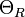$\Theta_R$ is the Debye temperature as obtained from resistivity measurements and matches very closely with the values of Debye temperature obtained from specific heat measurements. n is an integer that depends upon the nature of interaction:

1. n=5 implies that the resistance is due to scattering of electrons by phonons (as it is for simple metals)
2. n=3 implies that the resistance is due to s-d electron scattering (as is the case for transition metals)
3. n=2 implies that the resistance is due to electron-electron interaction.

As the temperature of the metal is sufficiently reduced (so as to 'freeze' all the phonons), the resistivity usually reaches a constant value, known as the residual resistivity. This value depends not only on the type of metal, but on its purity and thermal history. The value of the residual resistivity of a metal is decided by its impurity concentration. Some materials lose all electrical resistivity at sufficiently low temperatures, due to an effect known as superconductivity.

An even better approximation of the temperature dependence of the resistivity of a semiconductor is given by the Steinhart–Hart equation: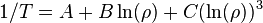$1/T = A + B \ln(\rho) + C (\ln(\rho))^3 \,$

where A, B and C are the so-called Steinhart–Hart coefficients.

This equation is used to calibrate thermistors.

In non-crystalline semi-conductors, conduction can occur by charges quantum tunnelling from one localized site to another. This is known as variable range hopping and has the characteristic form of$\rho = Ae^{T^{-1/n}}$, where n=2,3,4 depending on the dimensionality of the system.

## Complex resistivity

When analyzing the response of materials to alternating electric fields, as is done in certain types of tomography, it is necessary to replace resistivity with a complex quantity called impedivity (analogous to electrical impedance). Impedivity is the sum of a real component, the resistivity, and an imaginary component, the reactivity (analogous to reactance).

## Resistivity density products

In some applications where the weight of an item is very important, resistivity density products are more important than absolute low resistance. It is often possible to make the conductor thicker, to make up for a higher resistivity; and in that case, a low resistivity density product material (or equivalently a high conductance to density ratio) is desirable.

This knowledge is useful for long-distance overhead powerline transmission. Aluminum is used rather than copper because it is lighter for the same conductance. Calcium, while theoretically better, is rarely if ever used, due to its highly reactive nature.

Material Resistivity (nΩ•m) Density (g/cm^3) Resistivity - density product (nΩ•m•g/cm^3)
Calcium 33.6 1.55 52
Aluminum 26.50 2.70 72
Copper 16.78 8.96 150
Silver 15.87 10.49 166